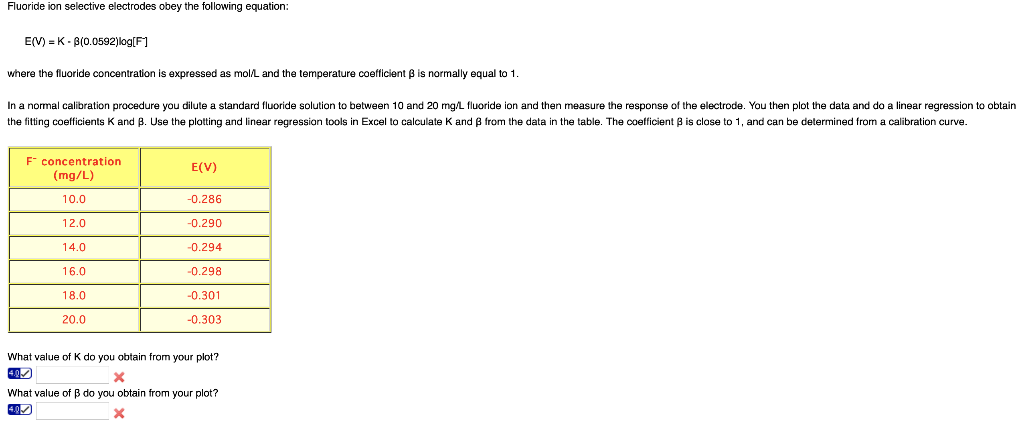Home / Answered Questions / Other / fluoride-ion-selective-electrodes-obey-the-following-equation-e-v-k-b-0-0592-log-f-where-the-fluorid-aw870

# (Solved): Fluoride Ion Selective Electrodes Obey The Following Equation: E(V) = K - B(0.0592)log[F] Where The ...Fluoride ion selective electrodes obey the following equation: E(V) = K - B(0.0592)log[F] where the fluoride concentration is expressed as mol/L and the temperature coefficient is normally equal to 1. In a normal calibration procedure you dilute a standard fluoride solution to between 10 and 20 mg/L fluoride ion and then measure the response of the electrode. You then plot the data and do a linear regression to obtain the fitting coefficients K and B. Use the plotting and linear regression tools in Excel to calculate K and from the data in the table. The coefficient is close to 1, and can be determined from a calibration curve. F concentration (mg/L) E(V) 10.0 -0.286 12.0 -0.290 -0.294 14.0 -0.298 16.0 18.0 20.0 -0.301 -0.303 What value of K do you obtain from your plot? What value of B do you obtain from your plot?

We have an Answer from Expert